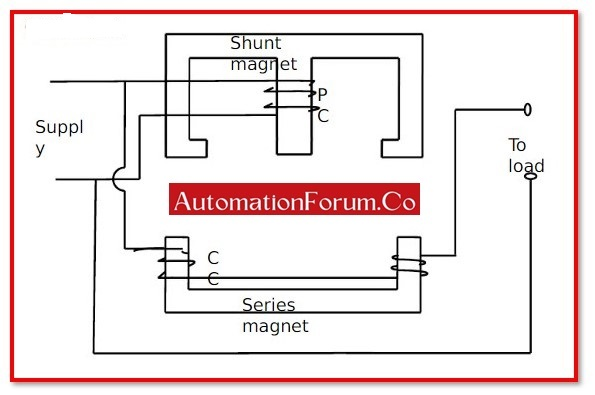# What is Wattmeter?A wattmeter is a device that measures the amount of electricity delivered to or generated by an electrical circuit. These instruments are frequently employed for the distribution and transmission of electric power, as well as for protection.

A wattmeter typically consists of two coils:

• Current coil and
• Potential coil

## What is Current Coil?

A coil, such as a wire wound around an electrical conductor, is essentially what a current coil is. Typically, a soft iron core acts as the conductor while insulated copper wire acts as the coil. It has been noted that the coil start functioning like a magnet when the conductor’s coil is carrying current.

## What is Potential Coil?

Potential coil refers to a coil or winding linked in shunt across a circuit (such as in a wattmeter). In order to carry a current that is proportionate to the supply voltage, a potential coil is attached across the supply line.

## What is the SI Unit of Wattmeter?

Watt is the SI unit for electrical power.

## Symbol of Wattmeter:

A circle on the capital letter W can be used to represent a wattmeter.

## How Wattmeter Works?

Voltage (V), current (A), power (W), power factor (pf), and energy consumption can all be measured with a conventional wattmeter (kWh). The range of measurement accuracy varies from 1% to 5% depending on the device’s brand and type. A wattmeter with a 2% measurement error can display a power of 100 W as 98 W, 102 W, or even 98 W. The equipment is measuring this ratio as it becomes down.

A lot of current can harm the wattmeter, which is a complicated instrument. All wattmeter is designed to handle a safe maximum current. The maximum permitted current in many devices is restricted to 16 A. It denotes a power output of 3,680 W at 230 V AC mains voltage.

It is important to keep this current and power value in consideration to avoid harming the wattmeter. Some gadgets also include overload protection.

It wouldn’t be inaccurate to imply that the wattmeter uses some energy as well. The typical power usage of a wattmeter is less than 0.5 W.

Thousands of times per second, the digital wattmeter measures the circuit’s current and voltage. With the aid of the internal computer, it multiplies the current and voltage to determine the electrical power.

Additionally, this processor is capable of recording data on lowest voltage, highest voltage, lowest power, and greatest power. It is possible to monitor the microprocessor, voltage changes, and power line interruptions.

## How to use a wattmeter:

The wattmeter is utilised in a variety of settings, including

• Laboratories,
• Industries, and
• Power distribution and transmission measurements.

## Watt and Volt:

The formula for the correlation between watt and volt is:

Where,

• Power is measured in watts.
• Electric potential is measured in volts.
• Current is measured in amperes.

The potential difference between two terminals of a conducting wire is measured in volts.

When a current of one ampere travels over a potential difference of one volt, the rate at which electrical work is produced is measured in watts.

A proportionate correlation was established between watt and volt. In other words, watt and volt are precisely proportionate. Which suggests that

• The electric potential in terms of volts increases as electric power in terms of watts increases, maintaining a constant electric current.
• Electric potential, measured in volts, decreases together with decreasing wattage, keeping electric current constant.

## Formula for Wattmeter:

The various electric circuits’ power is measured by wattmeter. It requires a power formula to work.

## Types of Wattmeter:

Following are two different types of wattmeter:

• Induction Type Wattmeter and
• Dynamometer Type Wattmeter

#### Induction Type Wattmeter:

• Shunt Magnet and
• Series Magnet

are the two laminated electromagnets that make up the induction wattmeter. The supply is connected across the shunt magnet, which conducts a current inversely proportional to the supplied voltage. The shunt magnet’s coil is designed to be very inductive, causing the current to lag the supply voltage by 90 degrees.

#### Dynamometer Type Wattmeter:

The operational field of a dynamo-type wattmeter, which uses moving coils, is created by a fixed coil elsewhere. A fixed coil with two equal parts that are parallel to one another makes up these instruments. To prevent hysteresis losses, these two fixed coils must operate together. The pointer is connected to the moving coil by a spring and begins to move when the moving coils begin to move as a result of an alternating current flowing through the fixed coil, which creates a magnetic field.